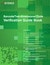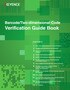1. Home >
2. ISO/IEC 15416 Verification Method

# ISO/IEC 15416 Verification MethodWithin the center area that makes up 80% of the barcode (excluding the top and bottom 10%),
10 scans worth of verification data are obtained every 8%.The grade of each scan is judged.
The range of grades is A, B, C, D, F.The grade of each scan is converted to a numeric value according to the table on the left.The average is calculated from the grades of the data from 10 scans. Then, this average is further classified into a grade according to the total grade judgment table.

In the example shown in the table on the left, the average is 2.9.The total grade is determined for the calculated average according to the table on the left.

In this example, the average is 2.9, so the grade is “B.”

Download